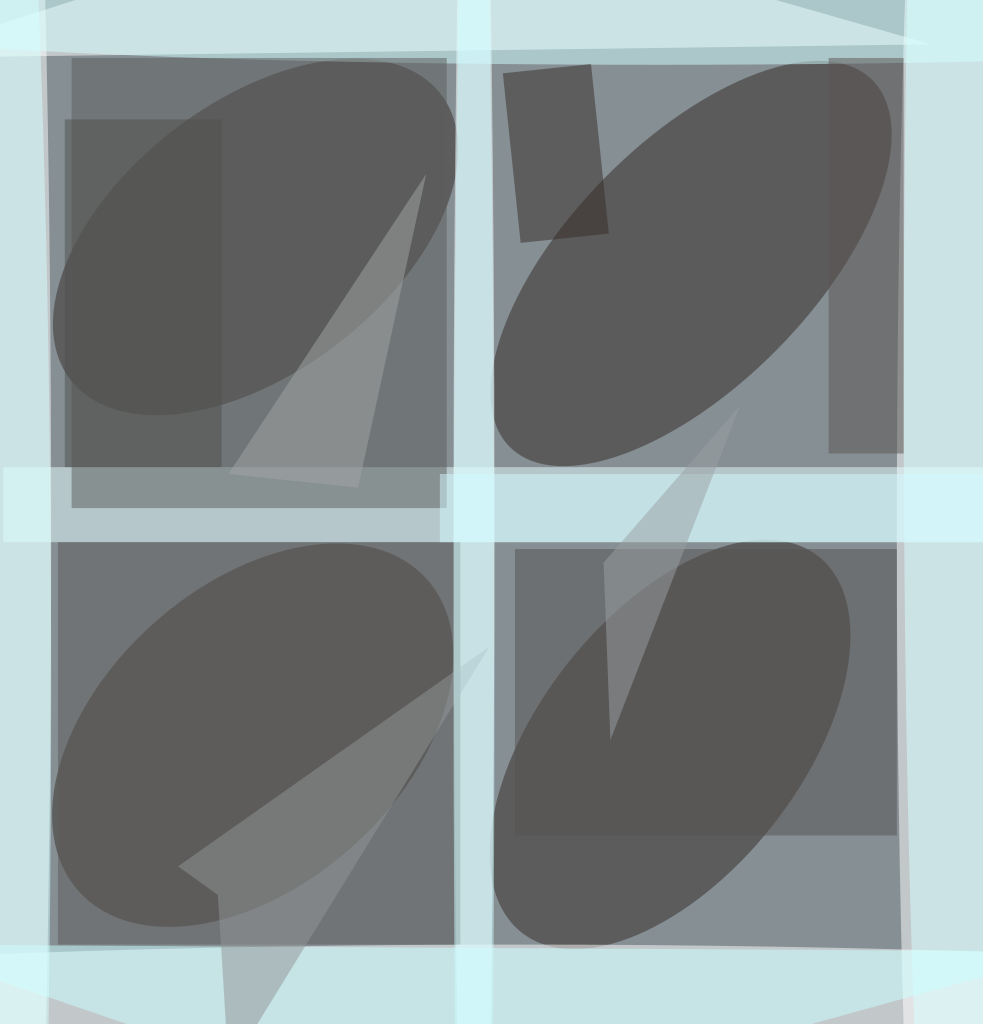A technique of partial subtractive dither has been developed to improve the performance of any of a variety of near-lossless data-compression algorithms. The technique may be applicable to compression of scientific and medical image data.To those skilled in the art of data compression, the use of subtractive dither to reduce the undesired quantization artifacts produced by lossy data-compression algorithms is well known. These artifacts include (1) biased average signal values in some regions of a signal, (2) steplike signal-value profiles in slowly changing portions of the signal, and (3) erasure of faint features that, if they occupied a sufficiently large area, would be detectable in the original signal.

The type of subtractive dither used heretofore, called "standard subtractive dither," involves a dither distribution that is uniform over a range equal to the quantization step size of the algorithm to which it is applied. Standard subtractive dither incurs costs in the form of an increase in rate (in other words, a reduction in compression) and an increase in distortion.

In the present technique of partial subtractive dither, the dither distribution is uniform over a range smaller than the quantization step size. The choice of a uniform distribution is motivated by a desire to optimally compromise between the benefit (reduction in quantization artifacts) and the costs (increases in rate and distortion) of standard subtractive dither. Under some reasonable assumptions, the dither distribution should be chosen to be uniform, with its range chosen according to the degree of dithering desired.

The figure shows the effects of compression followed by decompression of an image. As the degree of dithering increases from zero (no dither) through partial to standard, the appearance of streaks and artificial regions of constant intensity decreases, while the overall grainy appearance increases.

This work was done by Matthew Klimesh of Caltech for NASA's Jet Propulsion Laboratory. For further information, access the Technical Support Package (TSP) free on-line at www.nasatech.com/tsp  under the Information Sciences category.

NPO-20964

##### This Brief includes a Technical Support Package (TSP).